## Friday, January 04, 20192012
(November)
MATHEMATICS
(General)
Course: 101
[(a) Classical Algebra, (b) Trigonometry, (c) Vector Calculus]
Full Marks: 80
Pass Marks: 32
Time: 3 hours
The figures in the margin indicate full marks for the questions
GROUP – A
(Classical Algebra)

1. Answer the following questions: 1x4=4
1. The range of a real sequence may contain a complex number. (State True or False)
2. The elements of a real sequence can be put in a one-one correspondence with what set?
3. Every equation of odd degree has at least one real root. (State True or False)
4. Write the number of positive real roots of the equation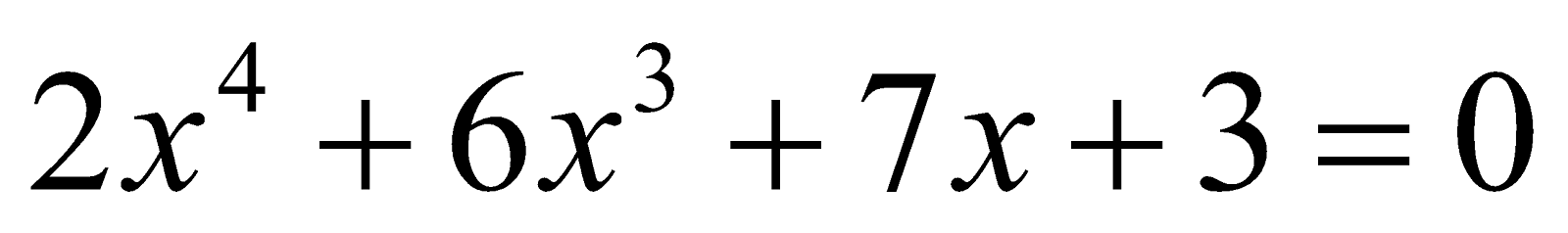2. Answer the following questions: 2x4=8
1. Write the limit point(s) of the sequence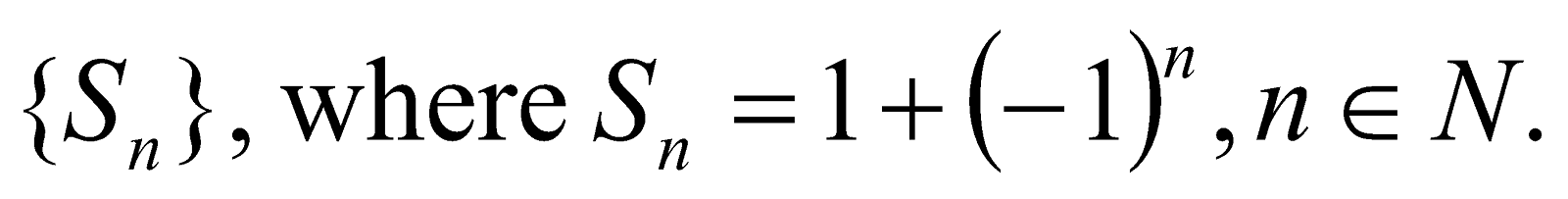2. Write the interval of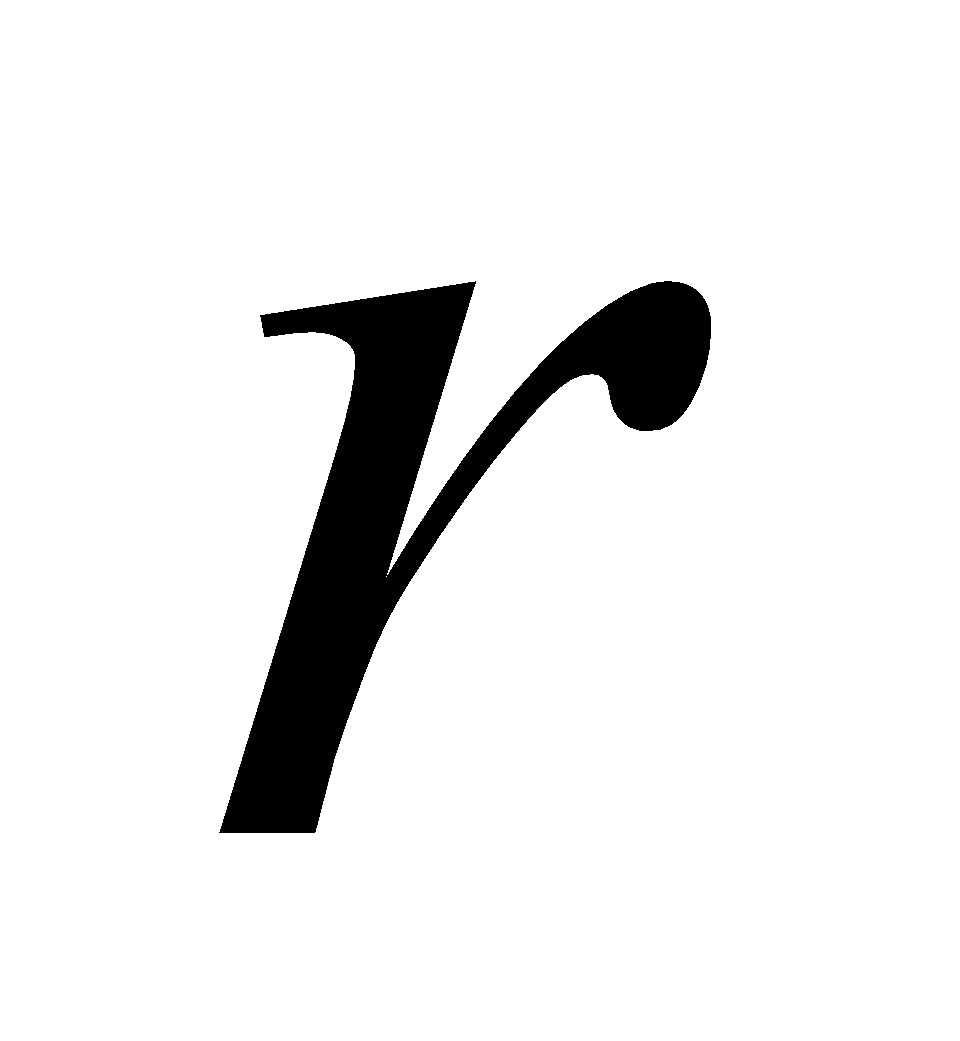for which the sequence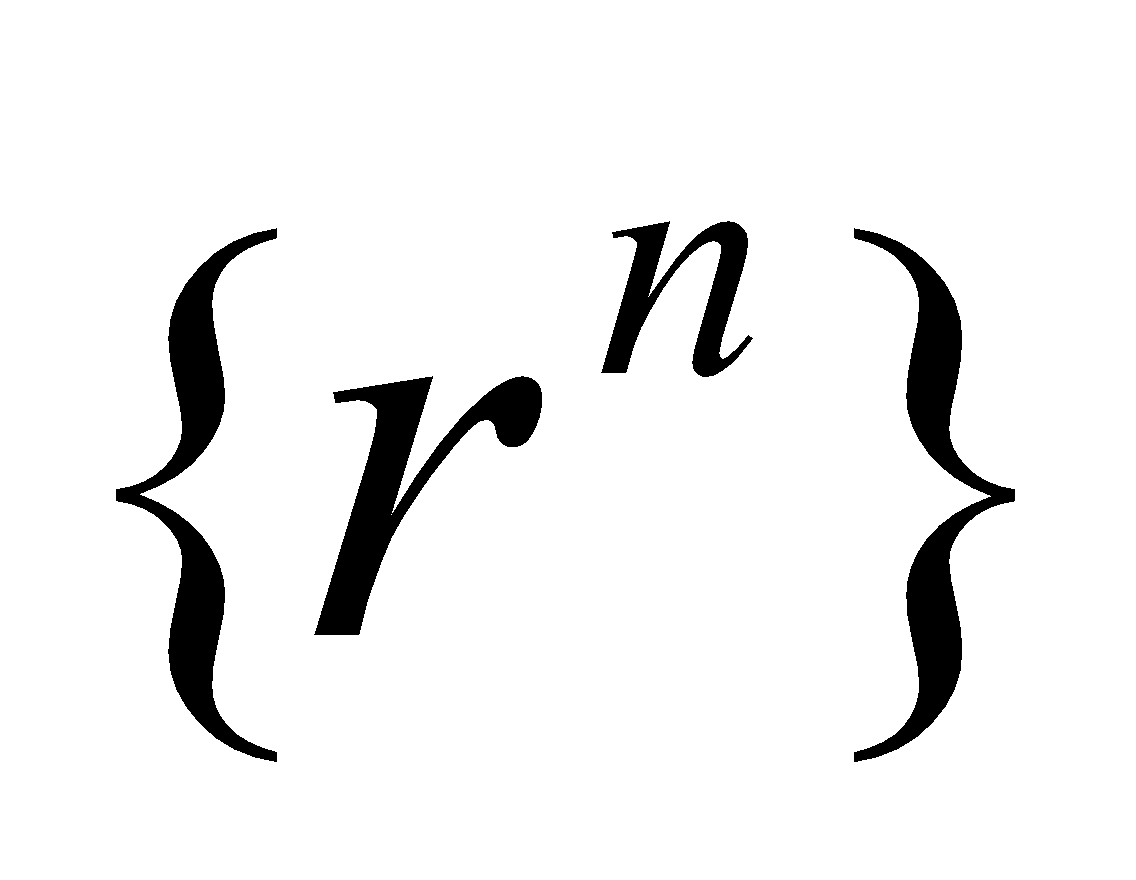converges.
3. Find the equation whose roots are the reciprocals of the roots of the equation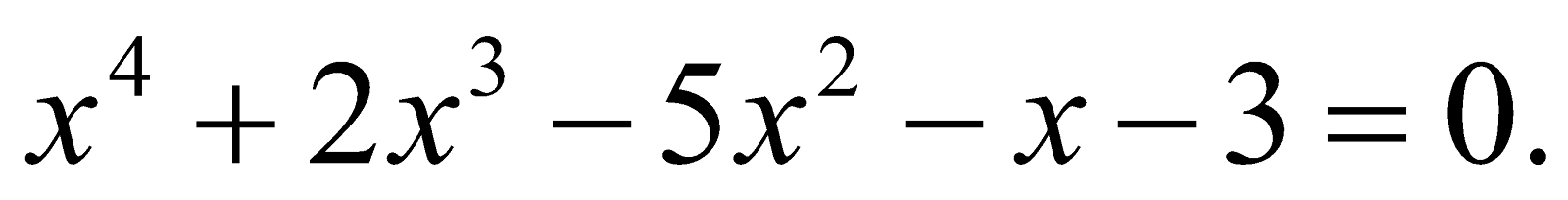4. Find the other root of the equation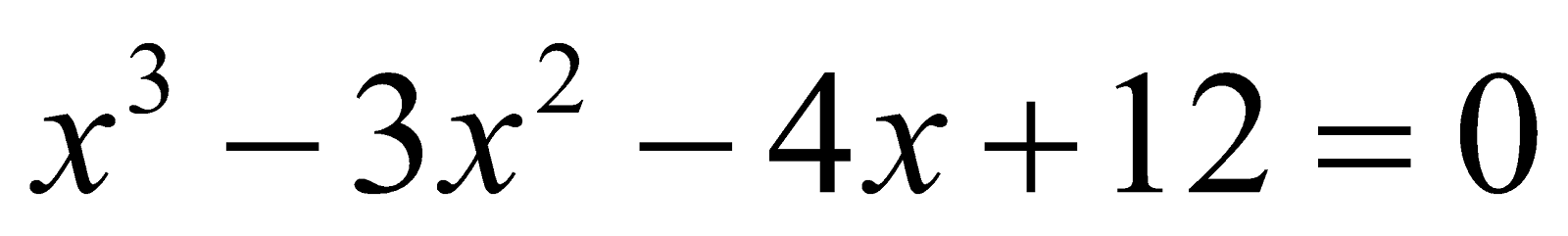whose two roots being equal in magnitude but opposite in sing.
3. Find the value of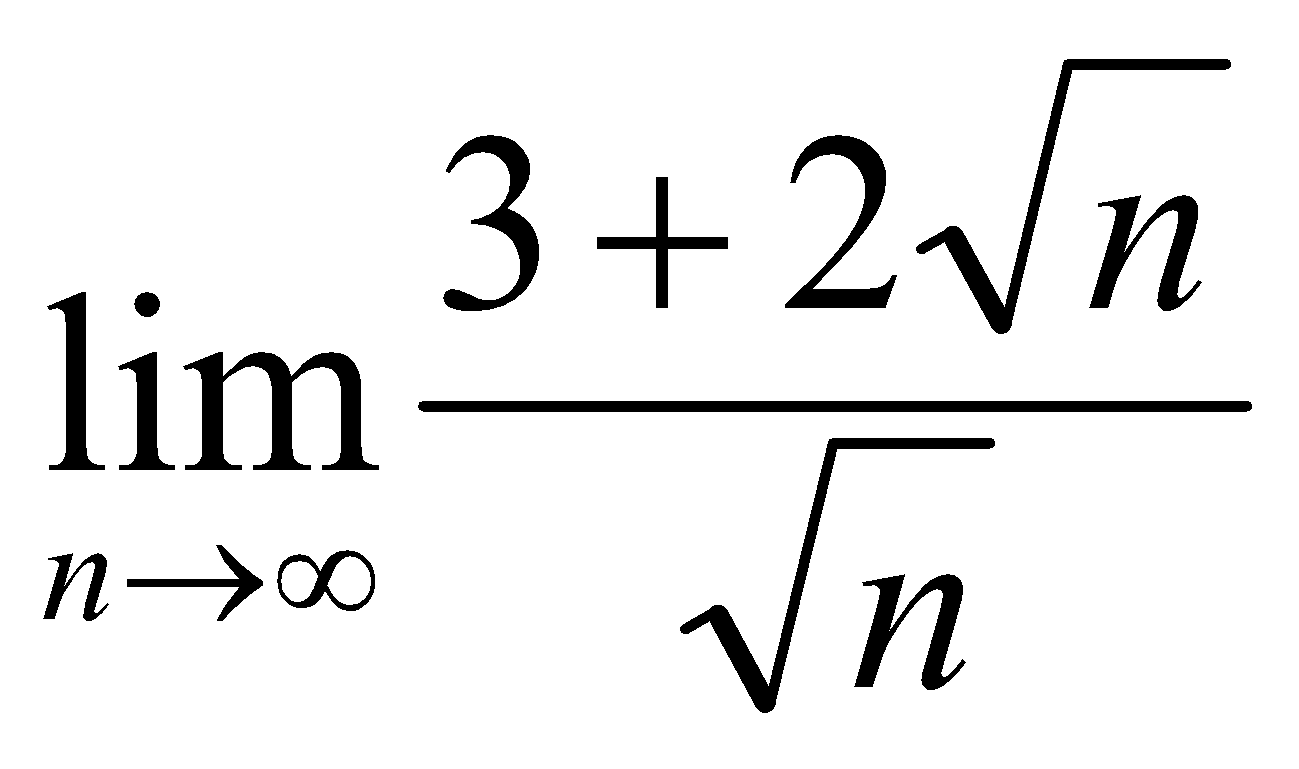3
4. Prove that every convergent sequence is bounded. 4
Or
Show that the sequence {1, 2, 3, 2, 5, 2, 7, 2, 3, 2, 11, 2, 13, …….}
oscillates infinitely.
5. Answer any two equations of the following: 5x2=10
1. Show that the series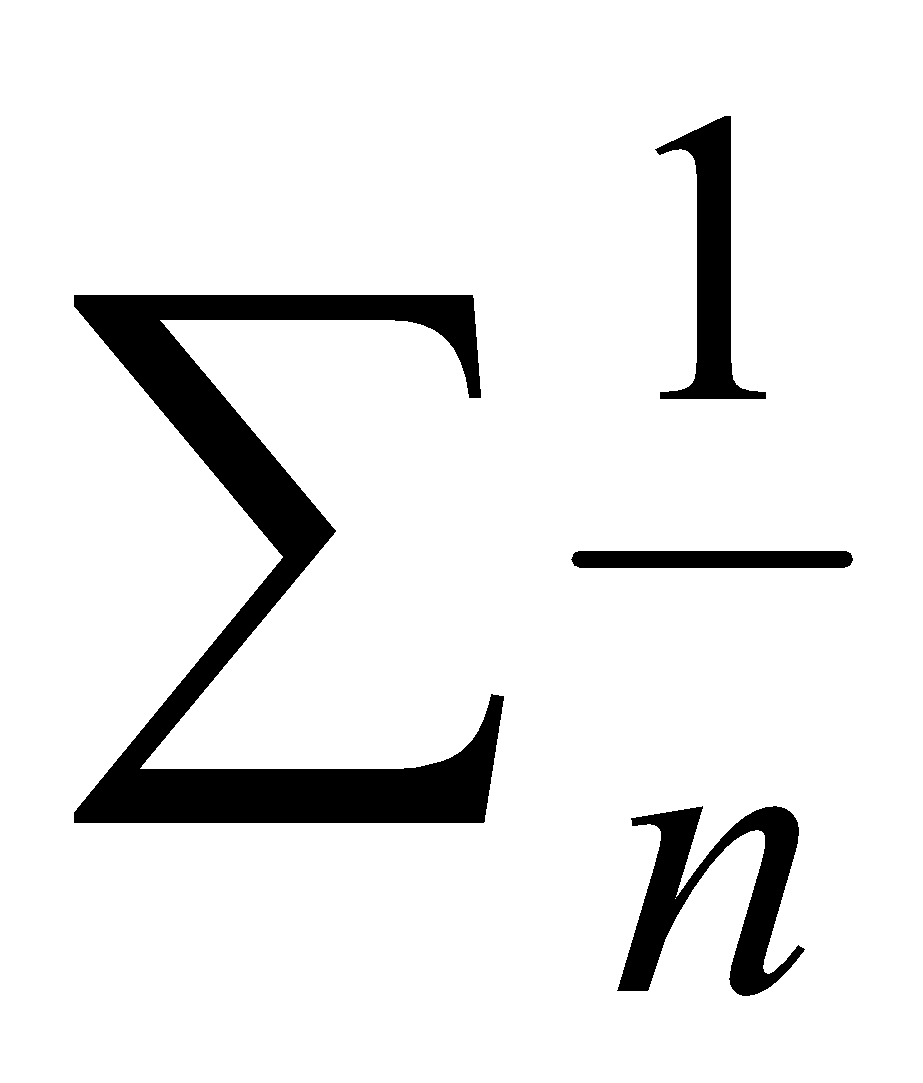does not converge.
2. Test the convergence of the series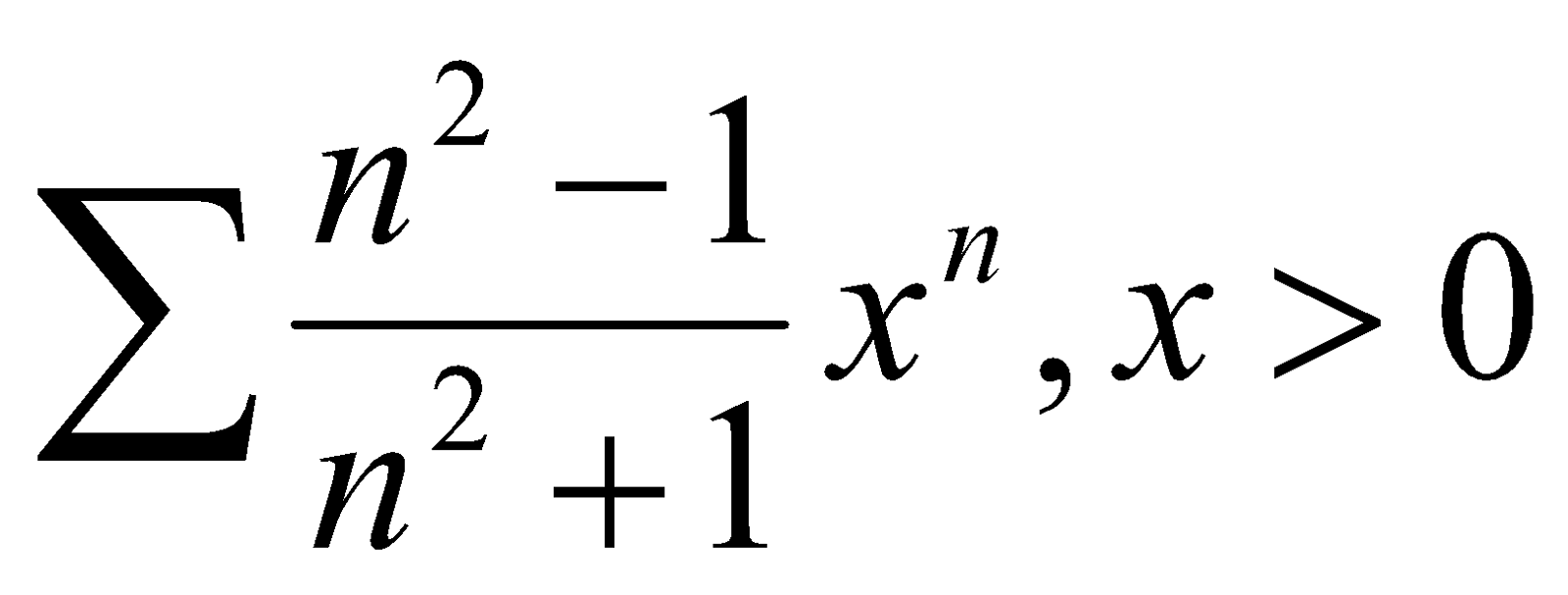3. Show that a series with positive term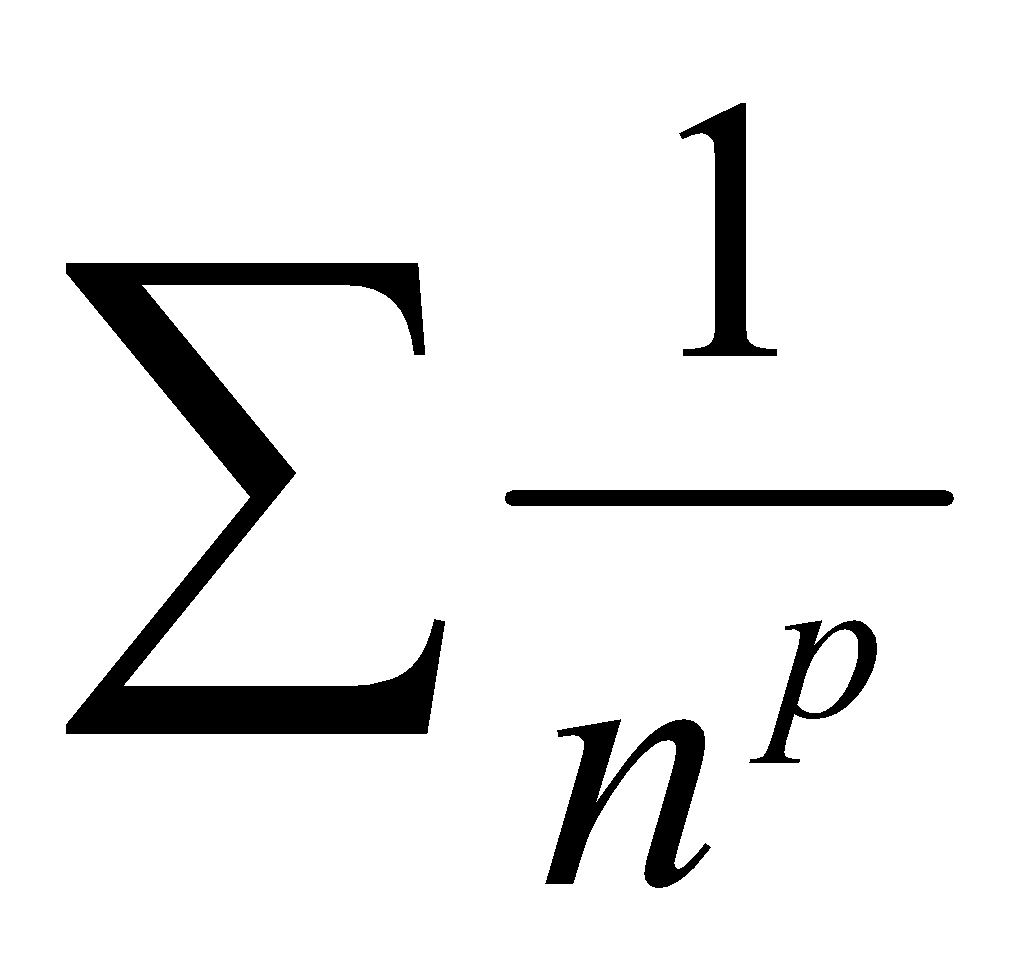is convergent for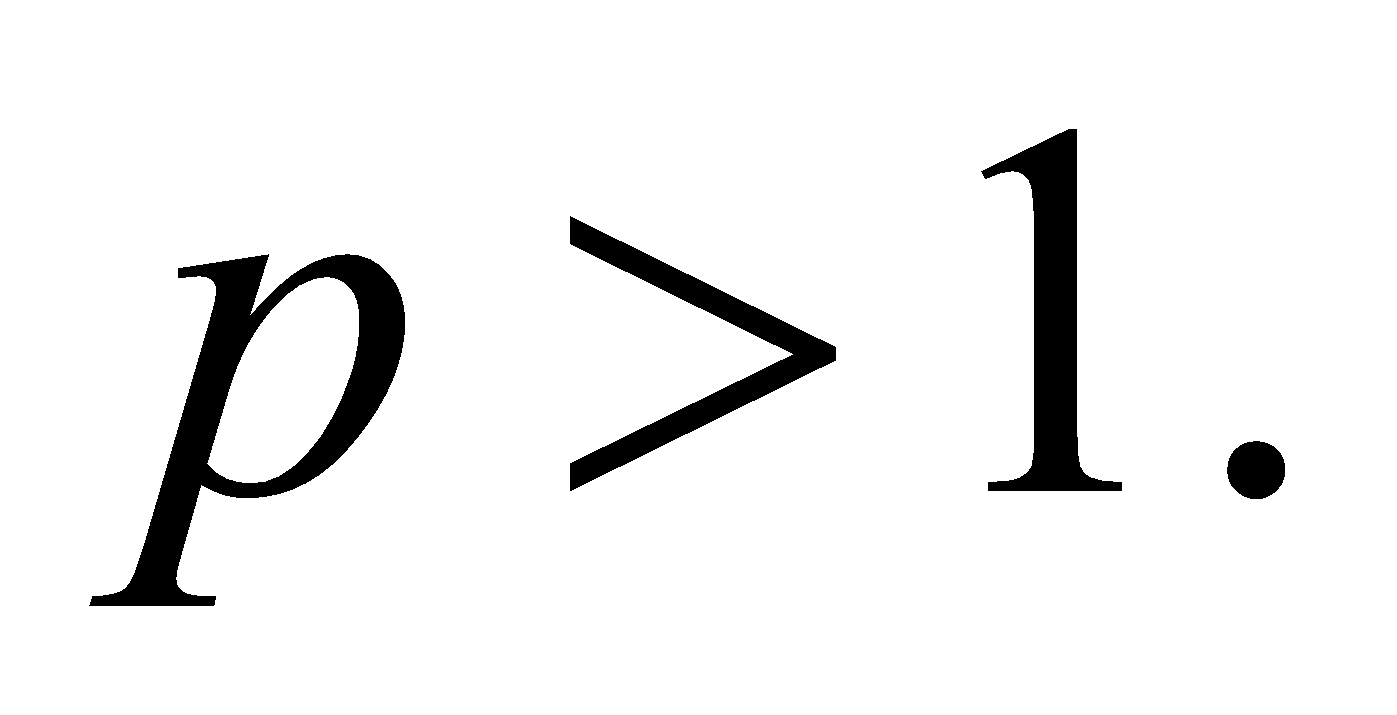6. Solve the equation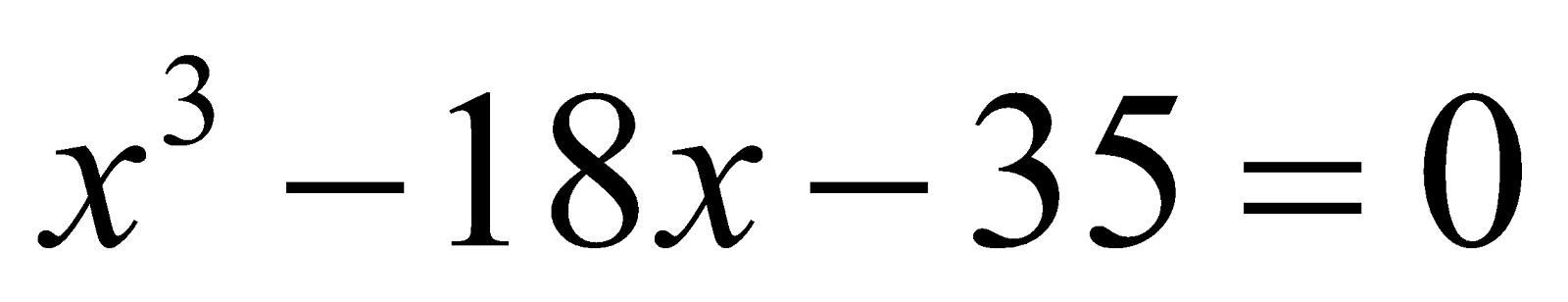using Cardin’s method. 10
Or
If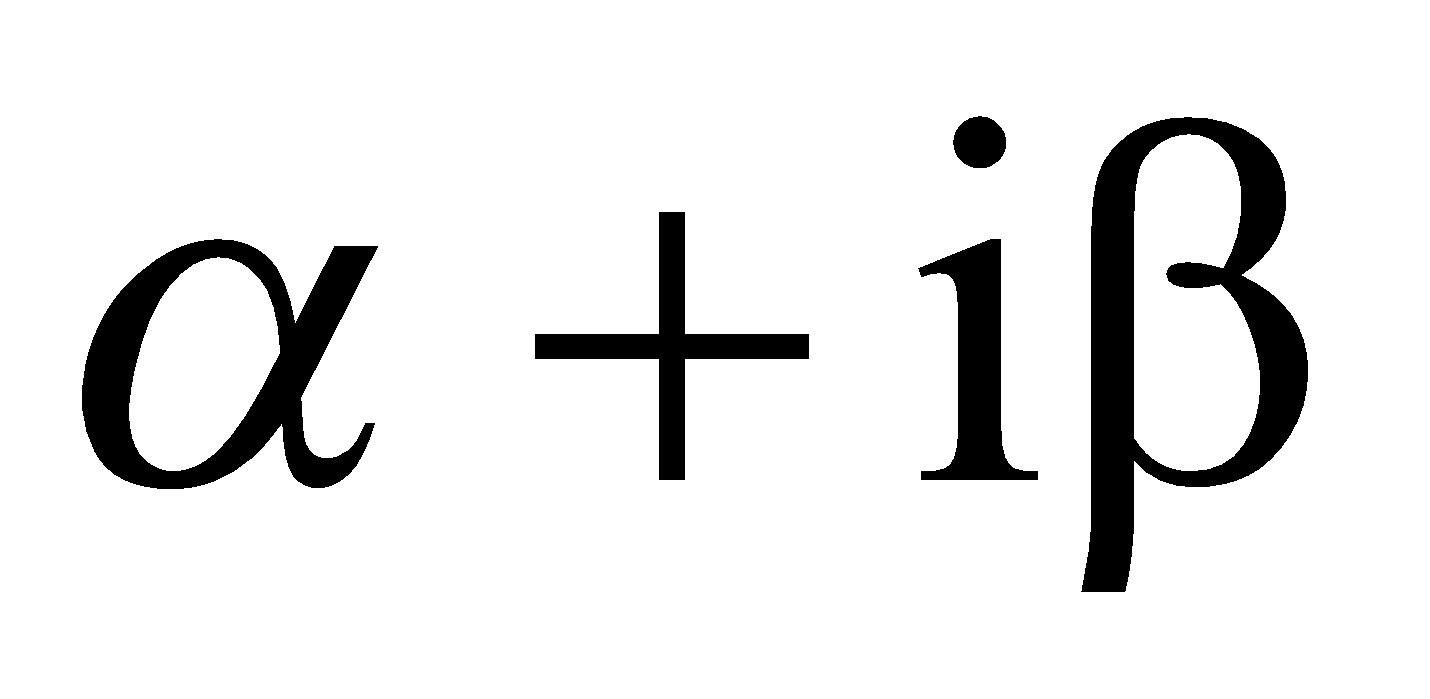be a root of the equation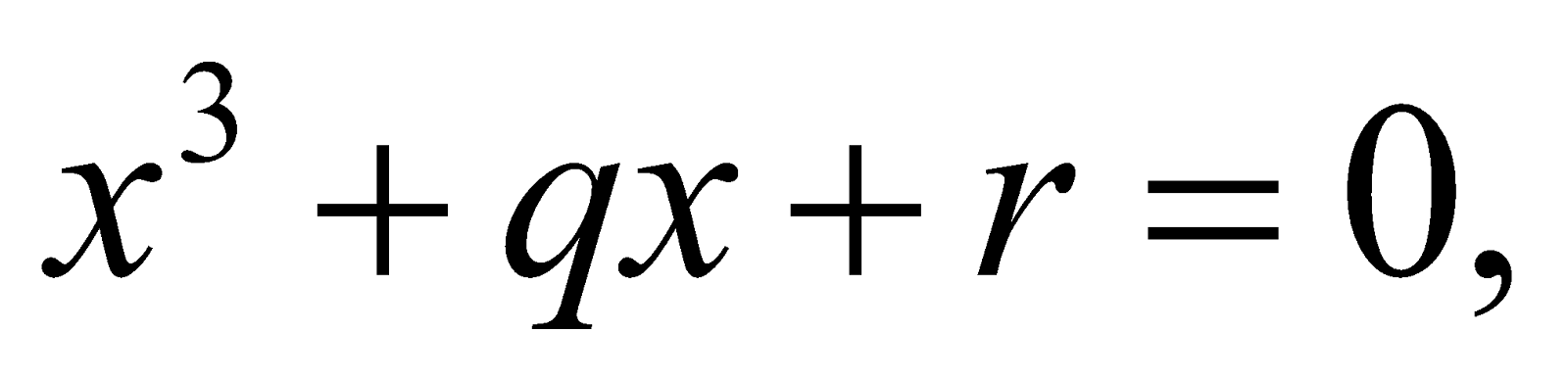then show that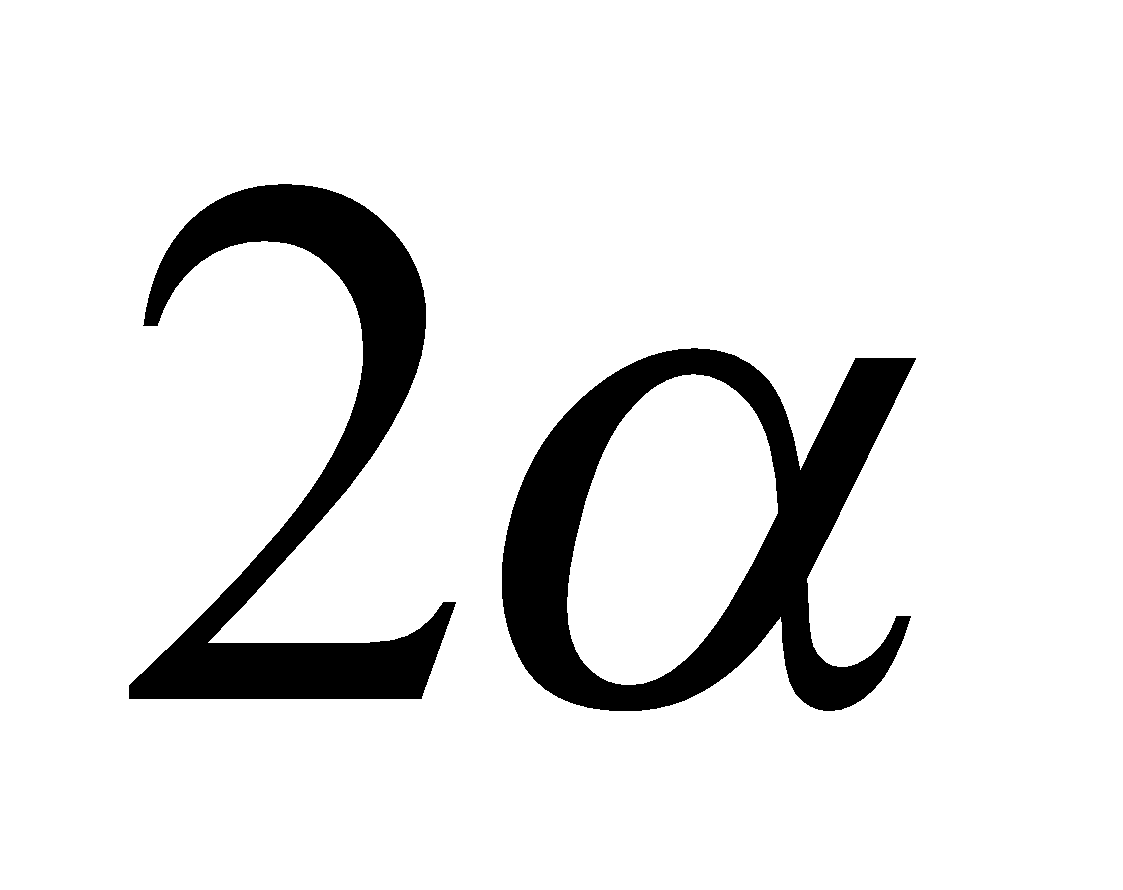is a root of the equation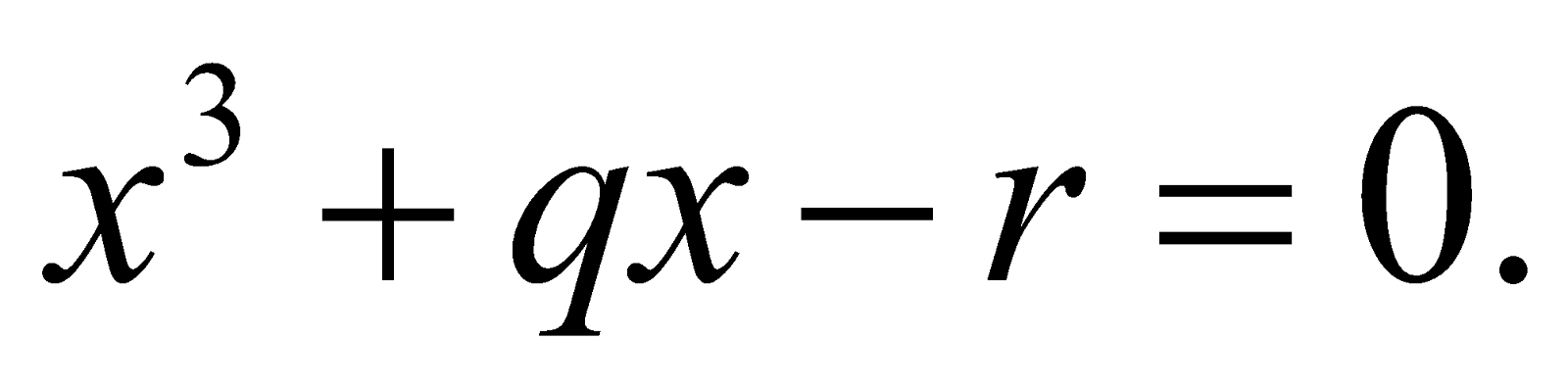7. Show that if a polynomial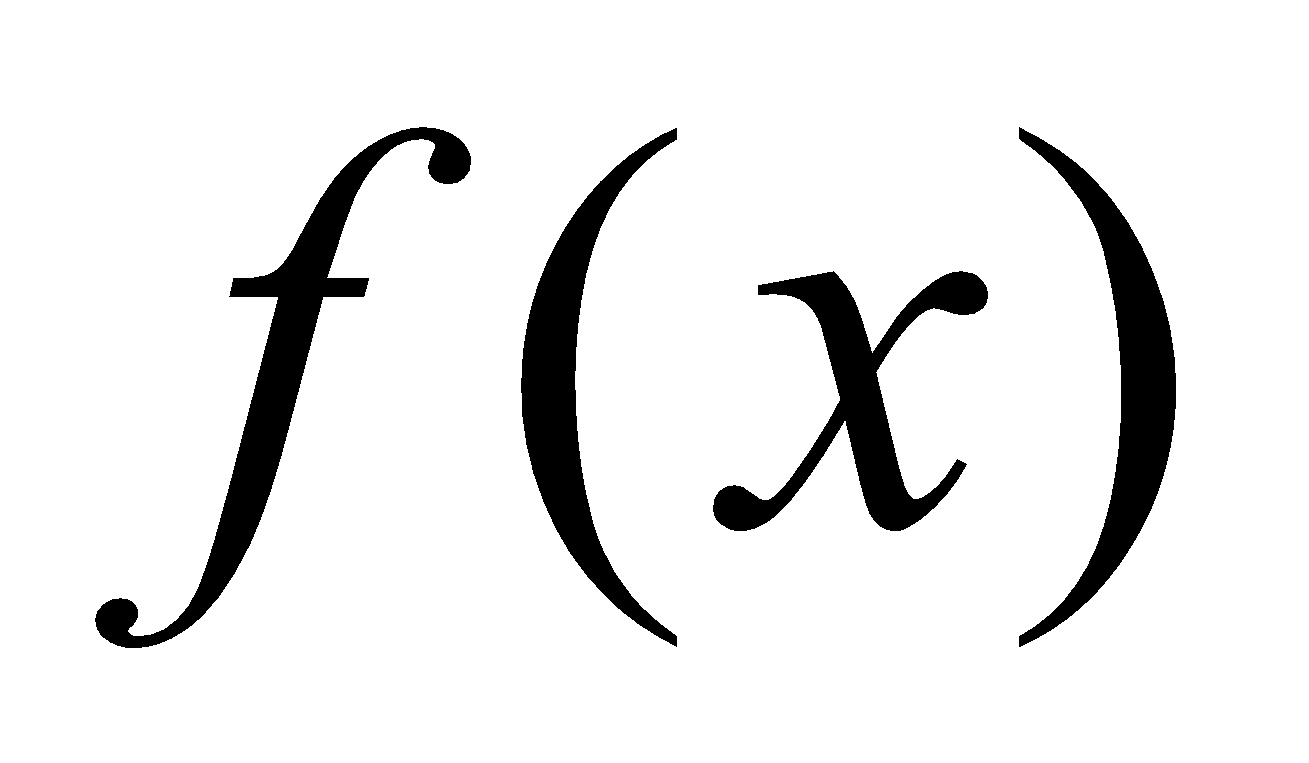be divided by a binomial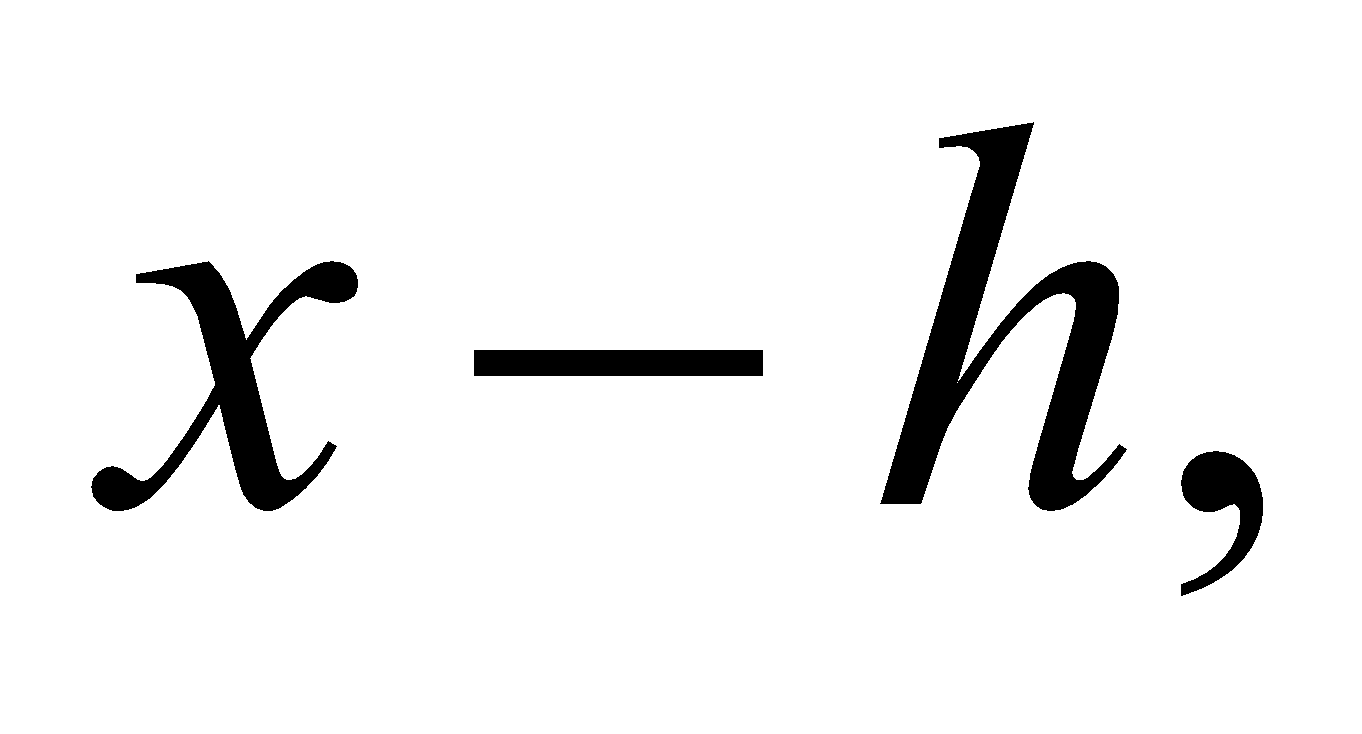then remainder is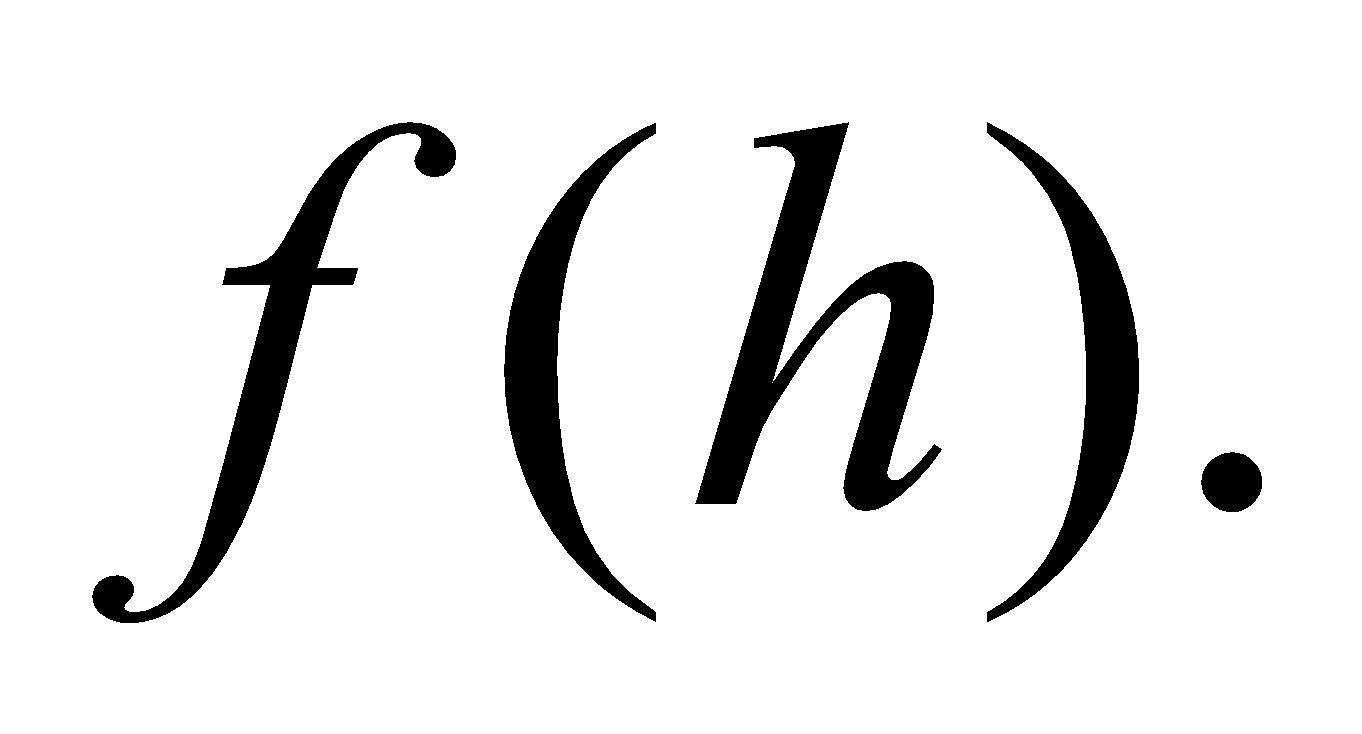5
GROUP – B
(Trigonometry)

8. (a) Write the solution (s) of the equation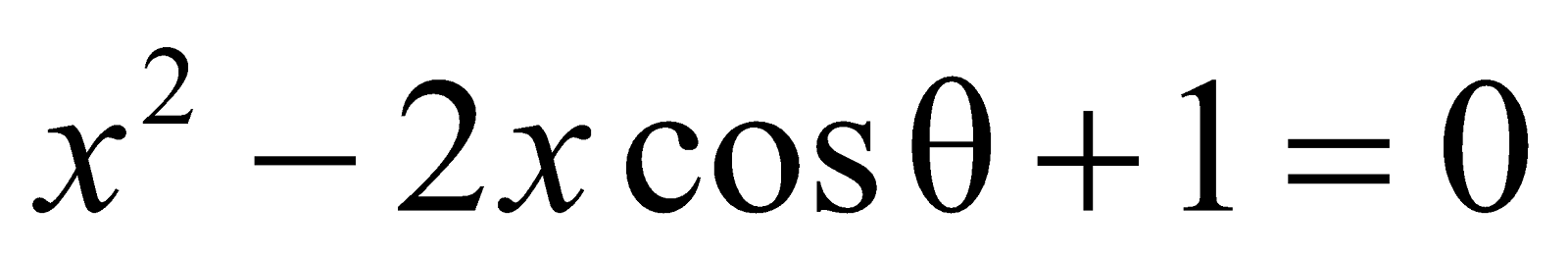1
(b) Write the number of values of logarithm of a complex number. 1
9. (a) Determine the value of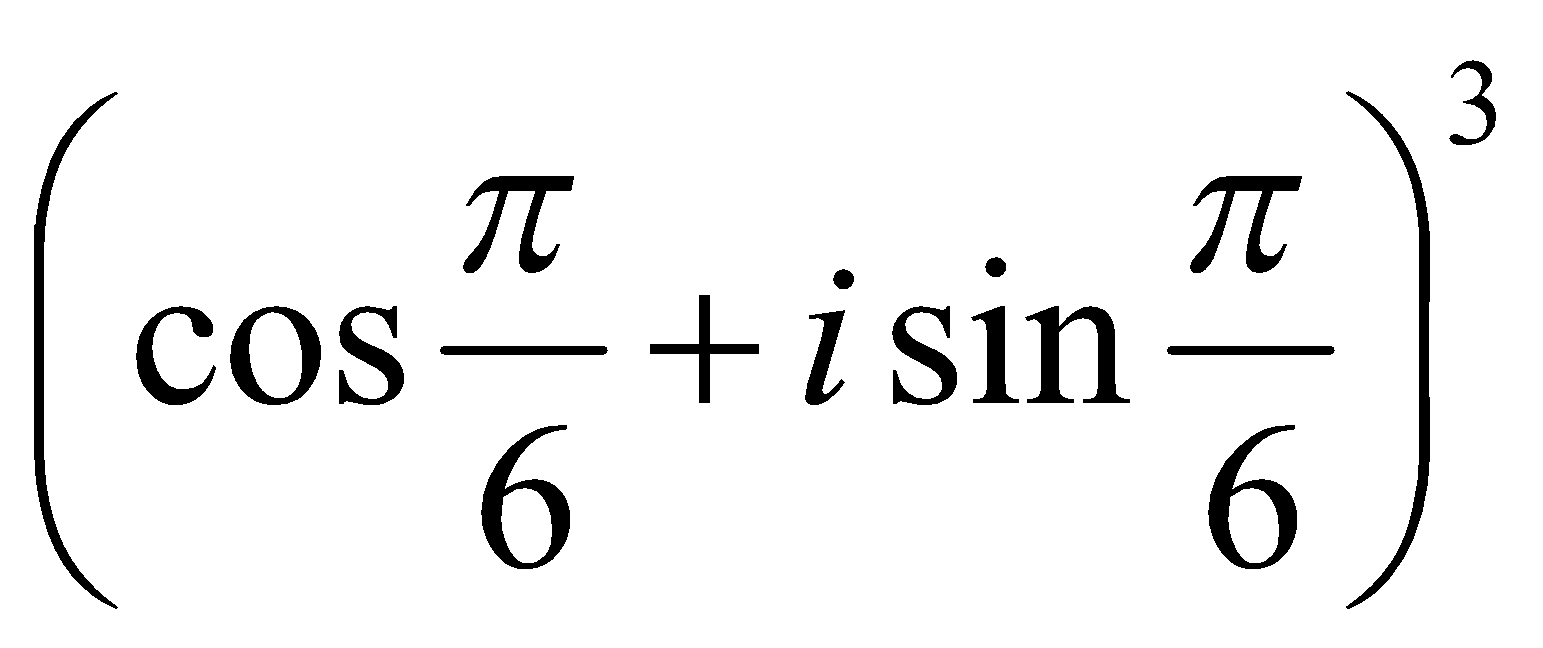(b) Write the sum of the series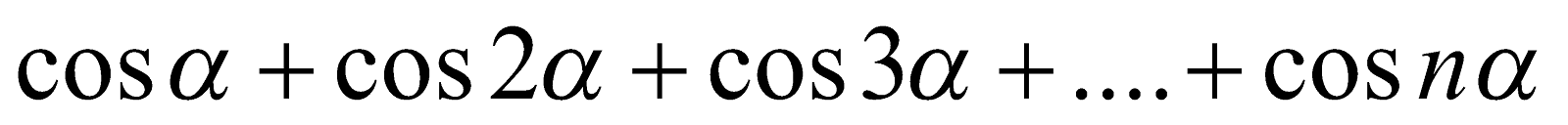. 2
10. If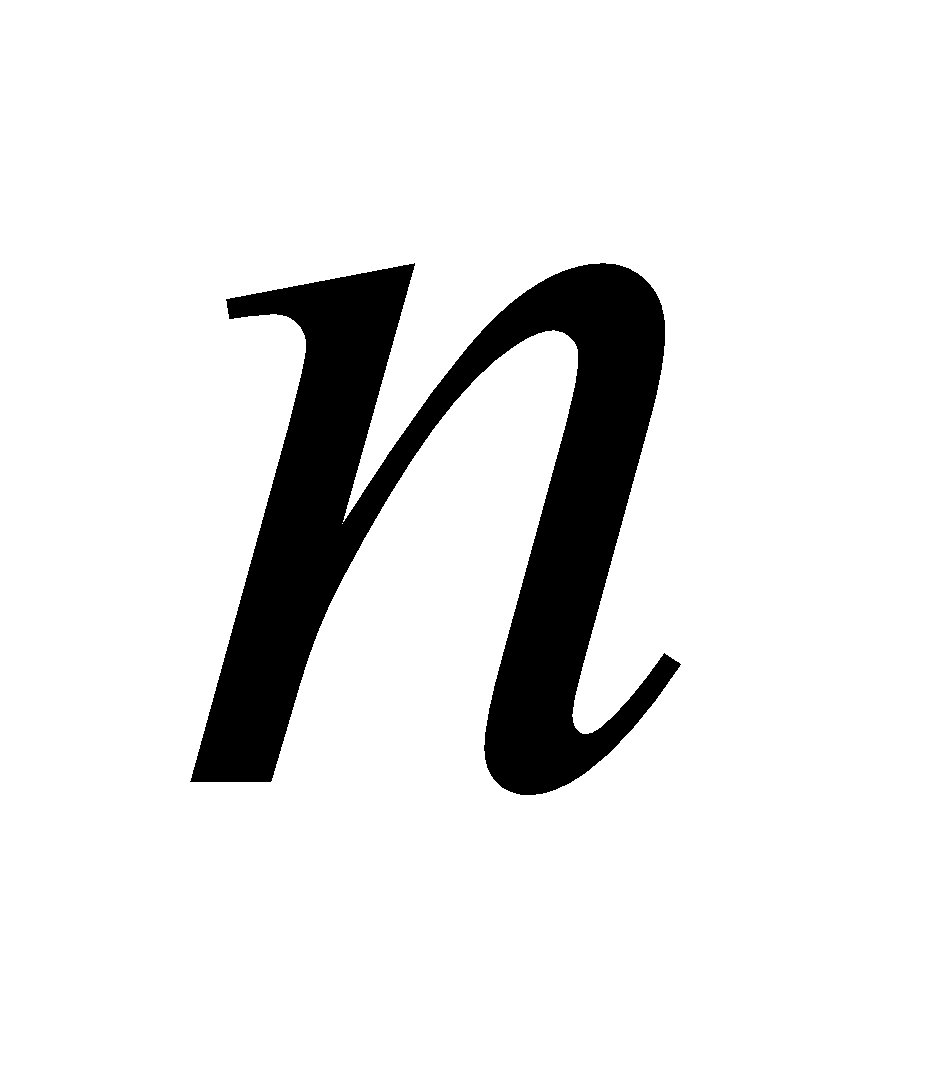is a positive integer, then show that 5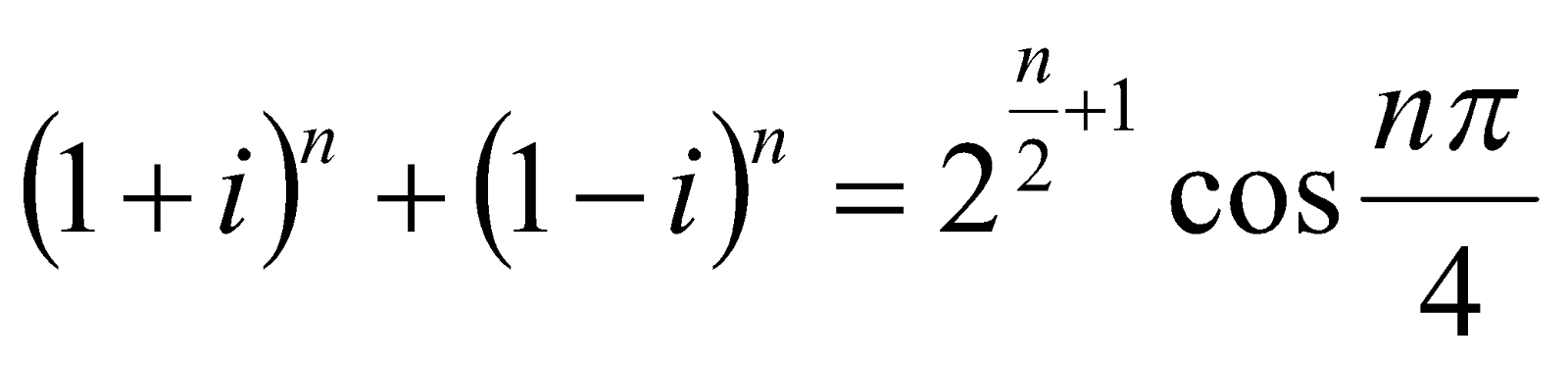Or
Find the value of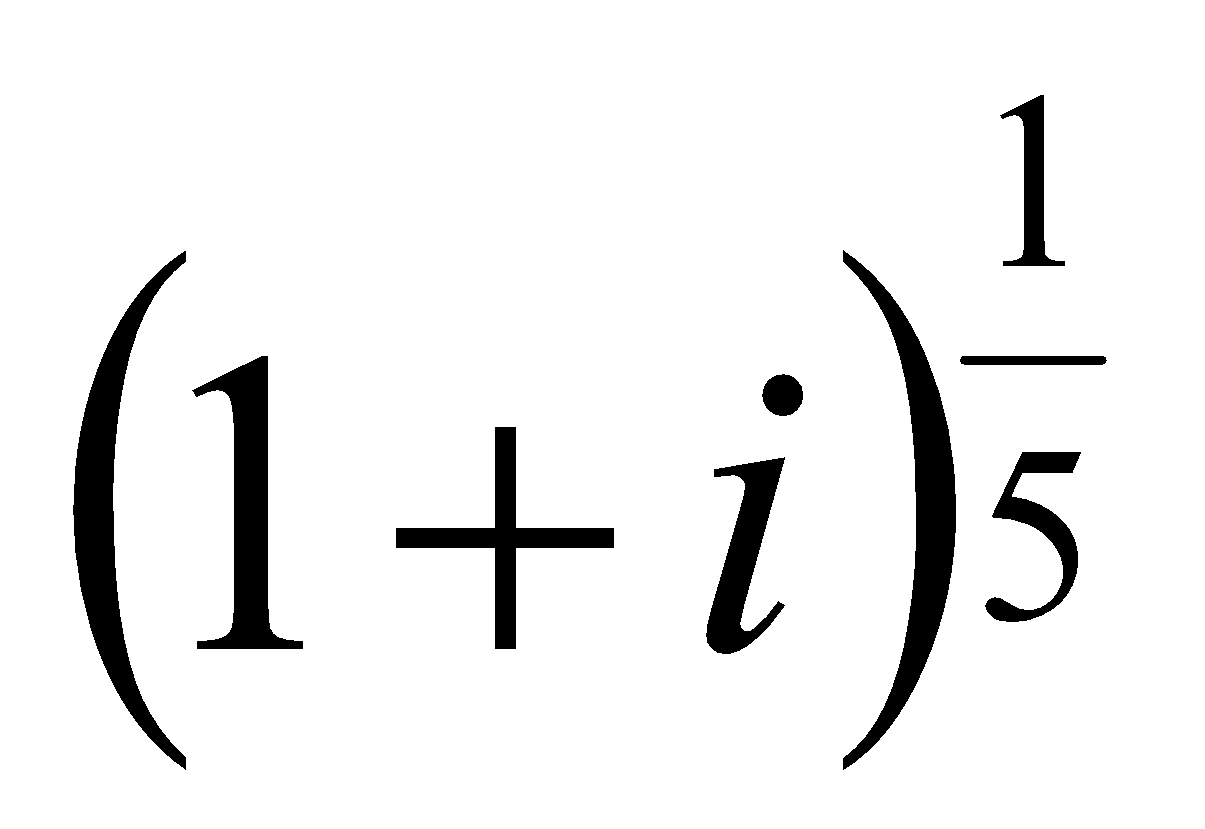11. Show that 4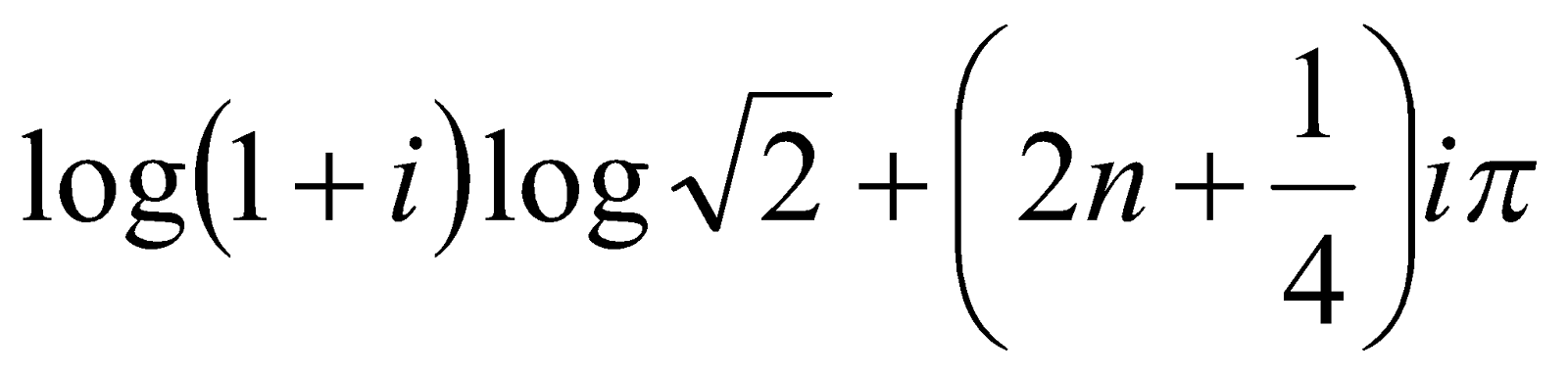Or
Show that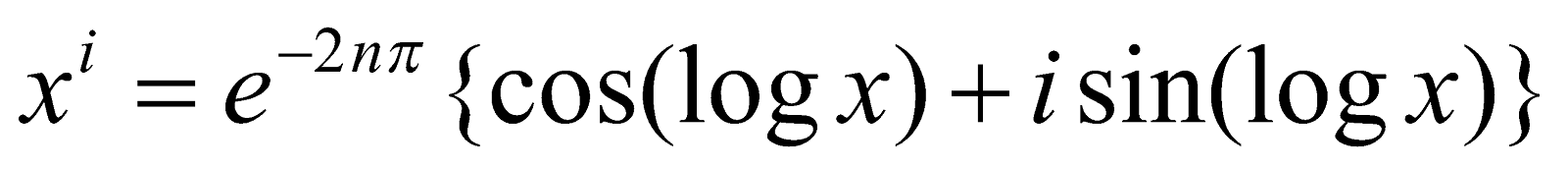12. Show that the coefficient ofin the expansion ofin powers of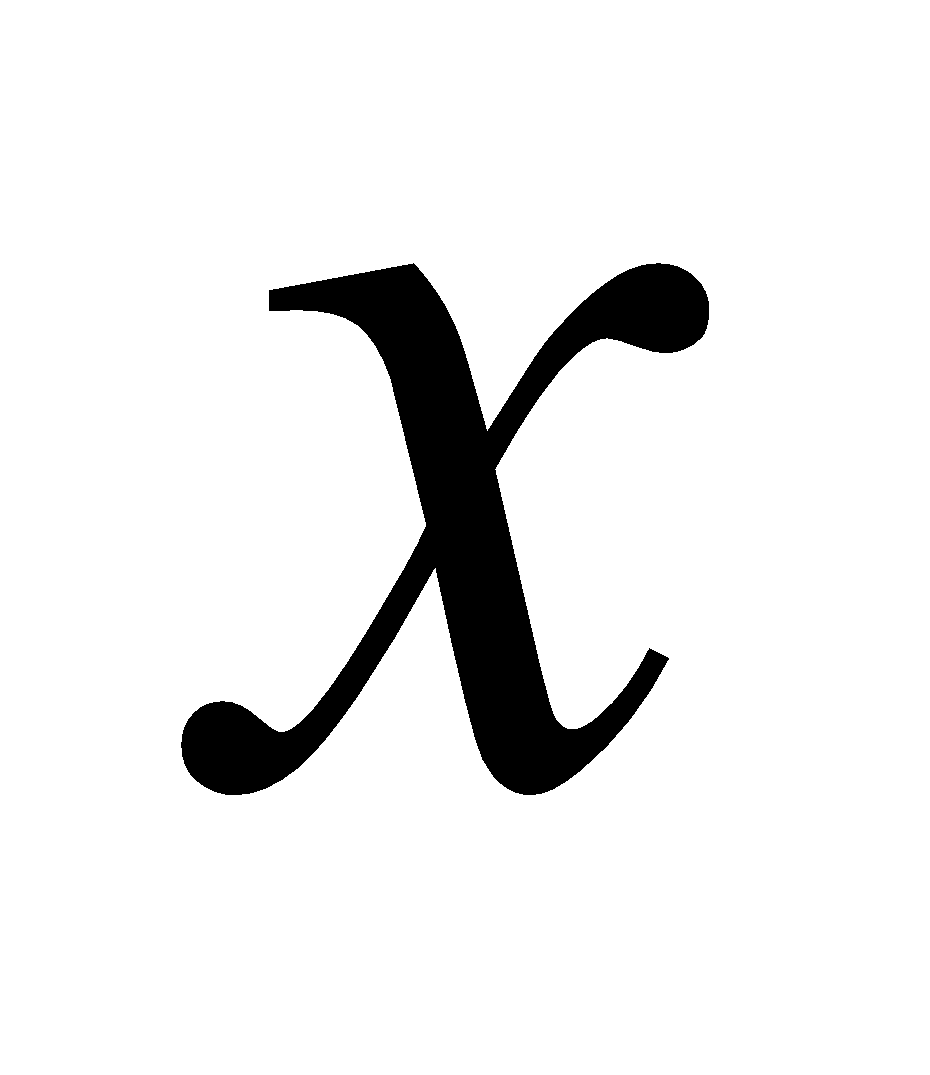is. 4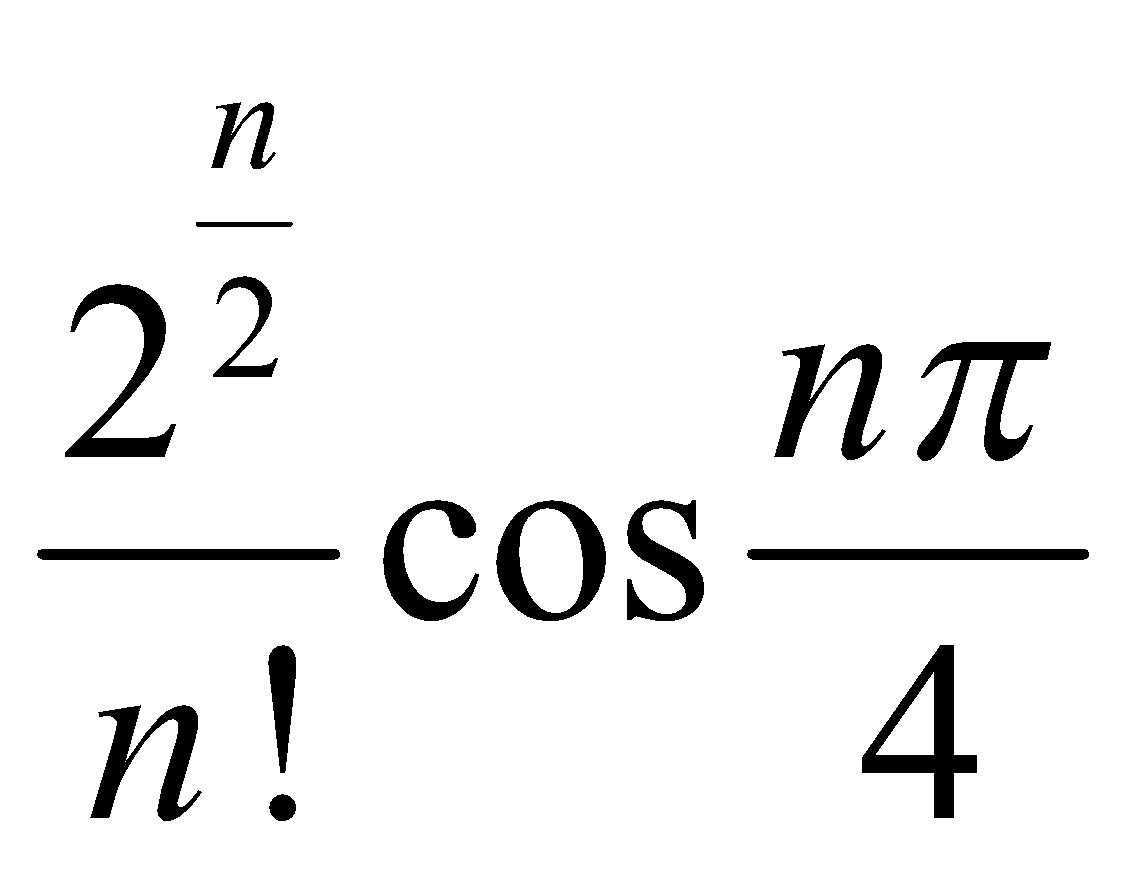13. Answer any two of the following: 3x2=6
1. Find the sum to n terms the series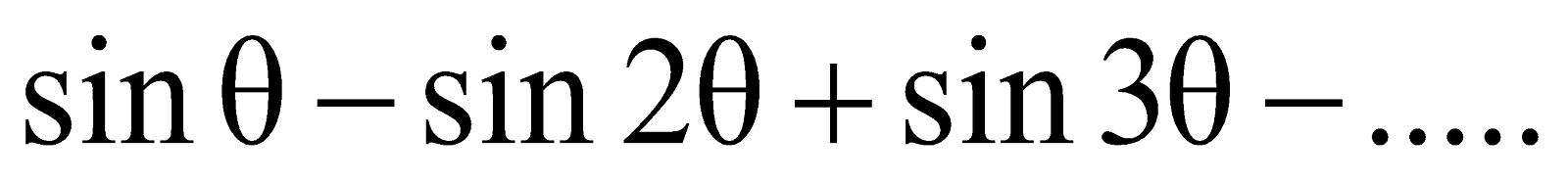2. Separateinto real and imaginary parts.
3. Prove that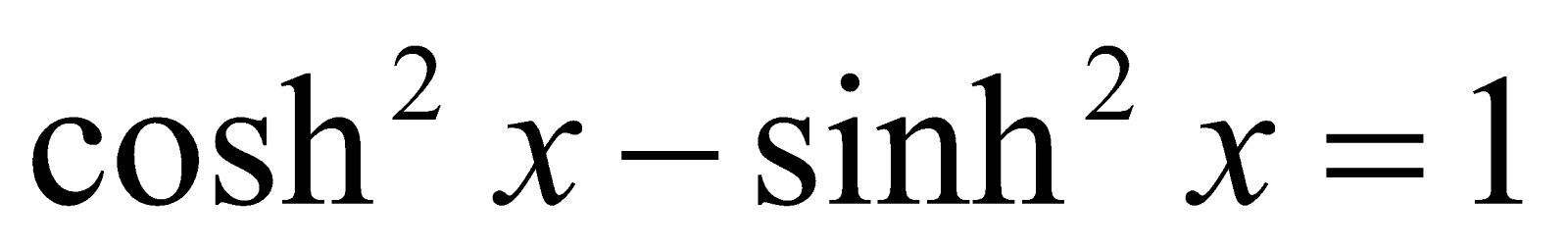GROUP – C
(Vector Calculus)

14. (a) Find the value of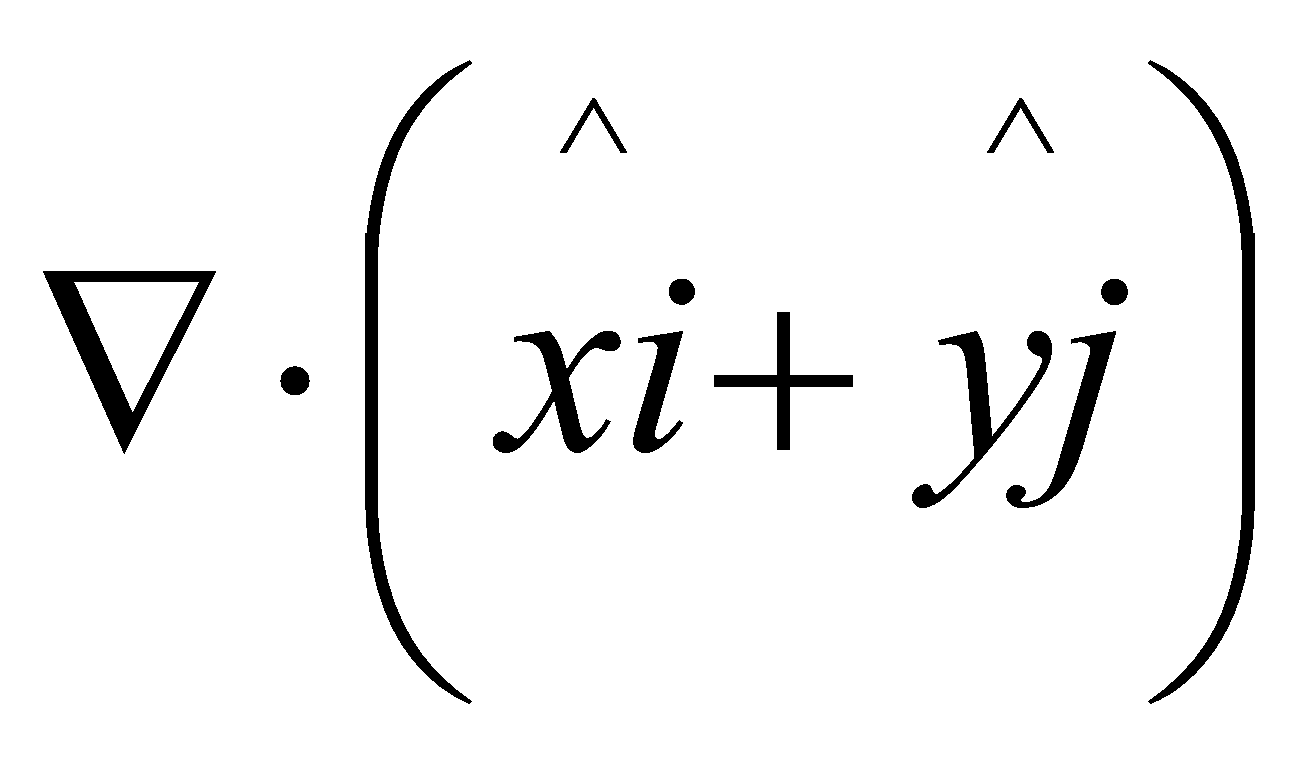1
(b) Write the definition of an irrotational vector. 1
(c) Find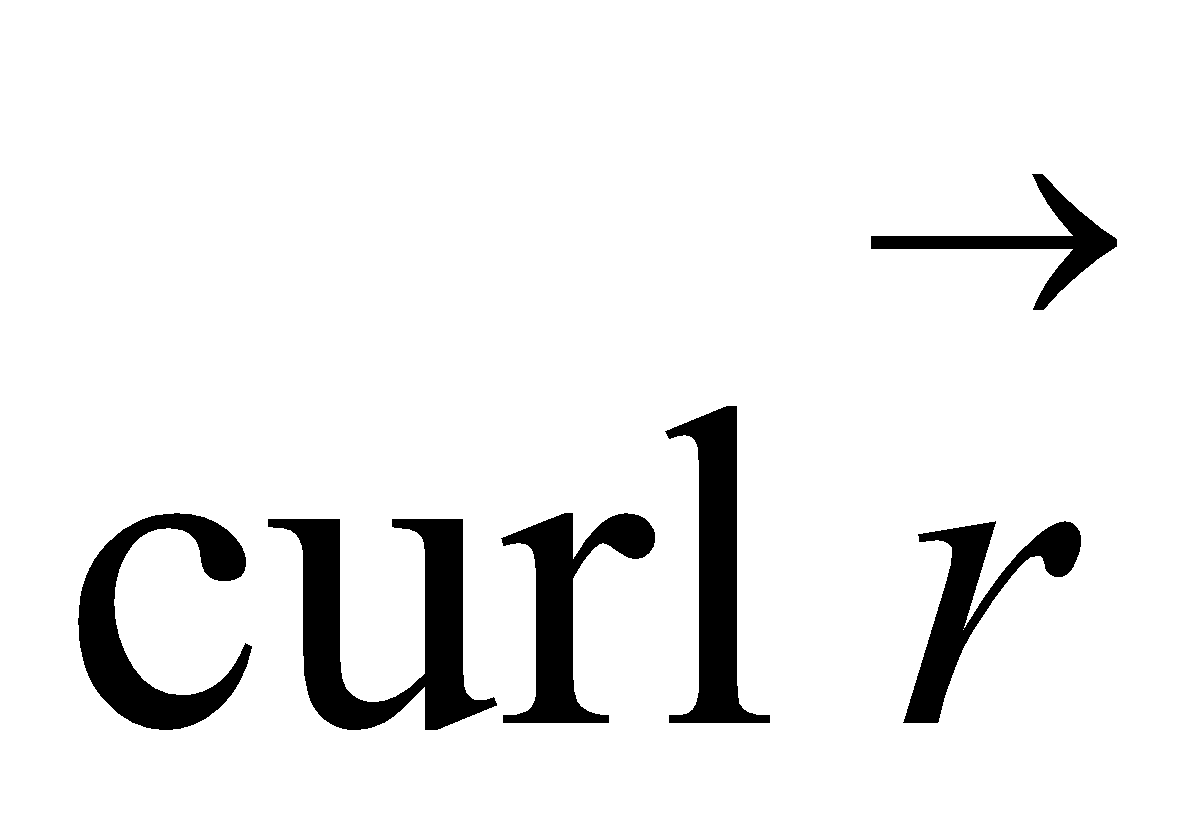, where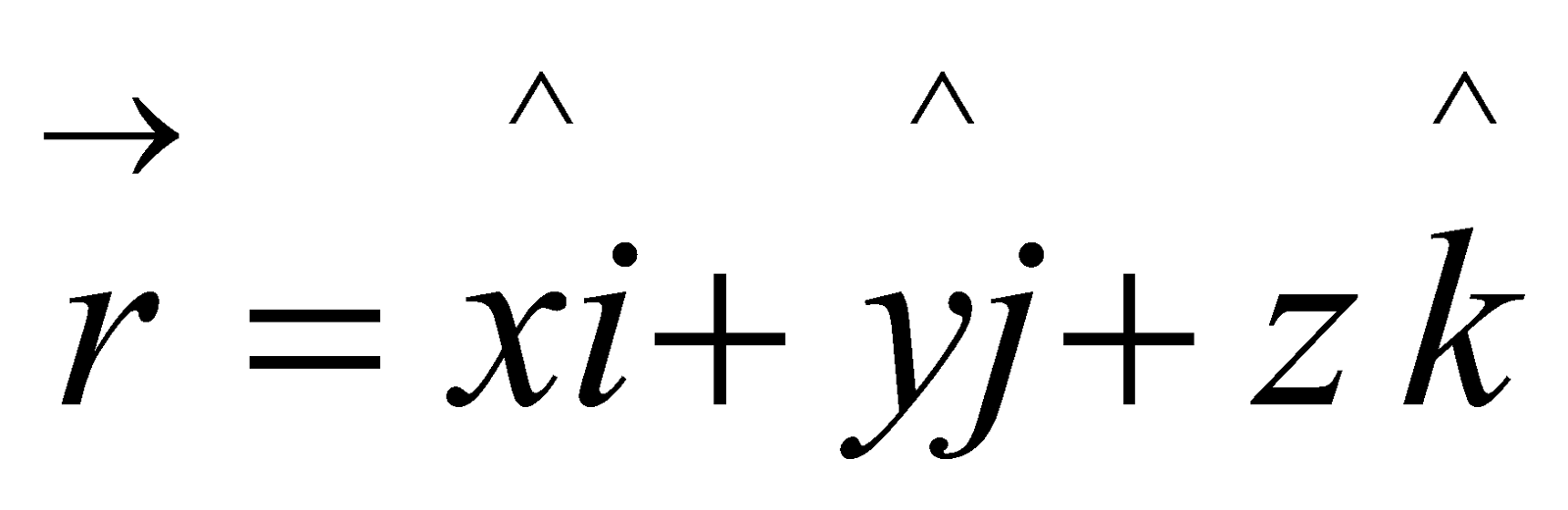2
15. Prove that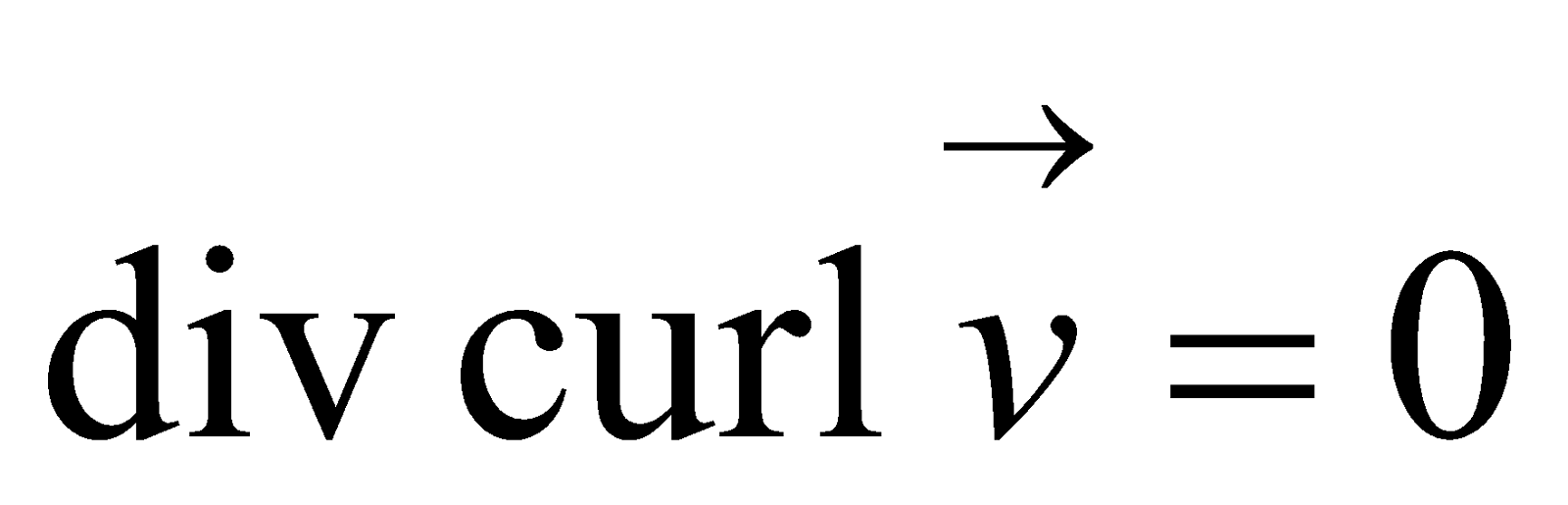3
16. Answer any two of the following: 4x2=8
1. Find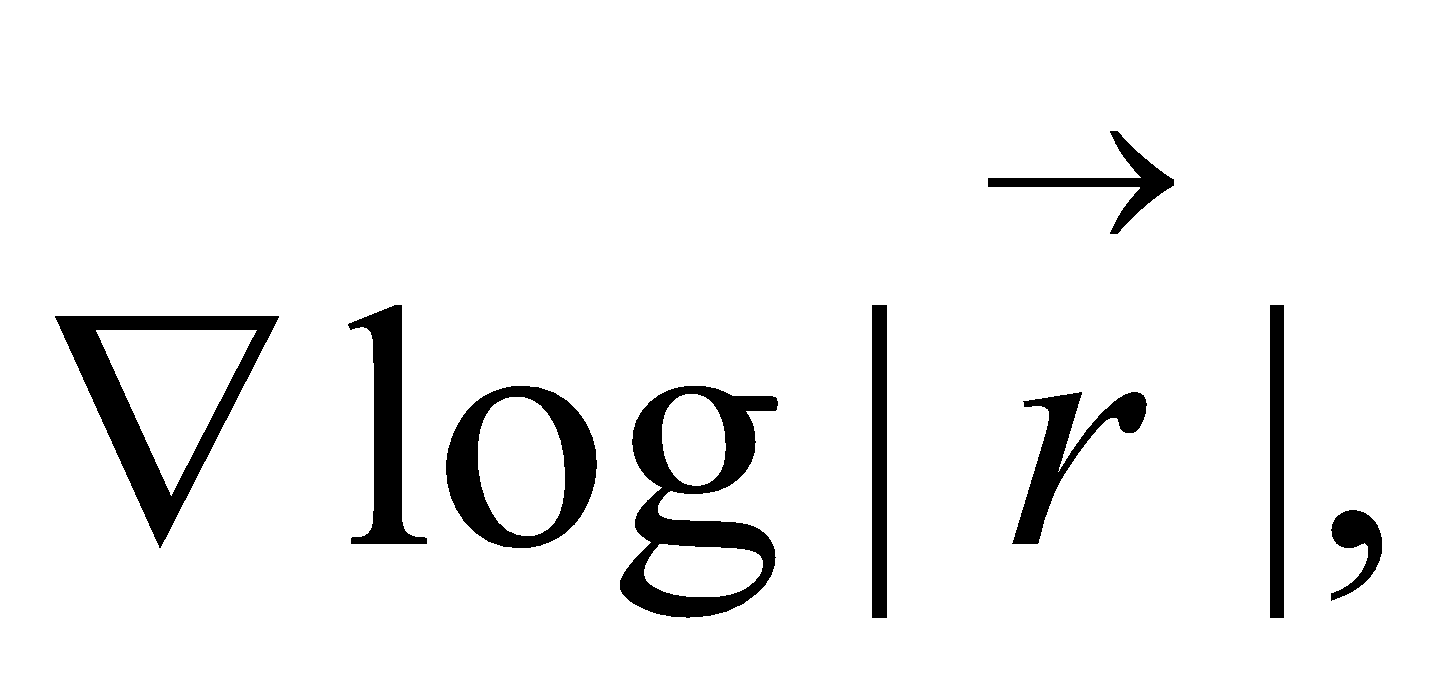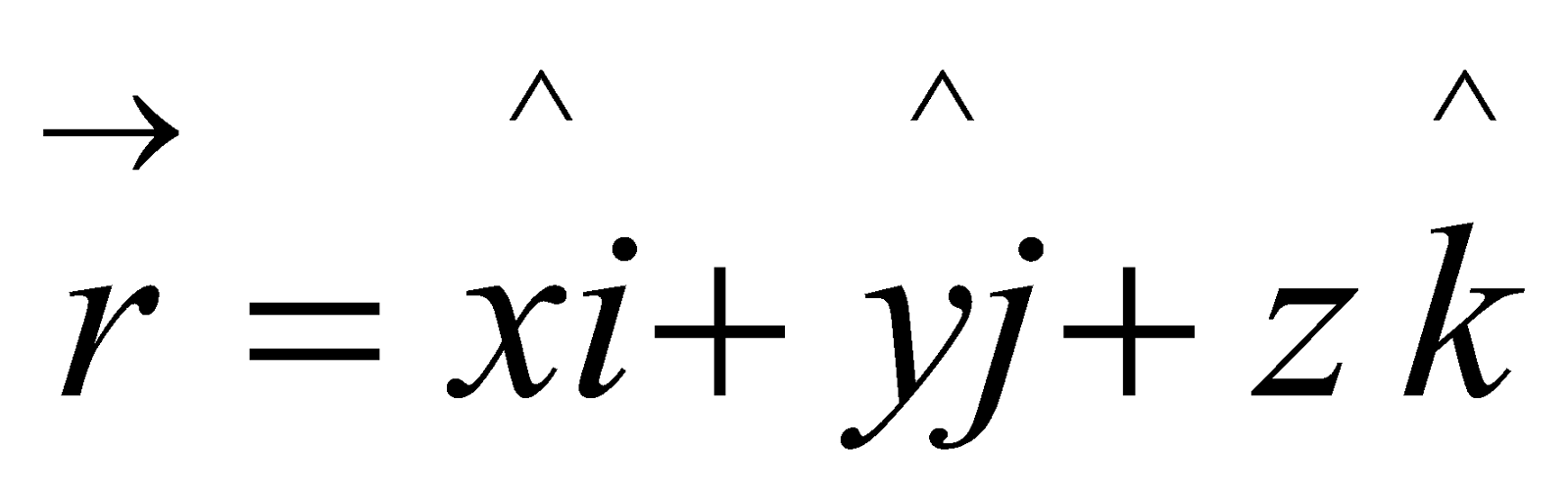2. Evaluate: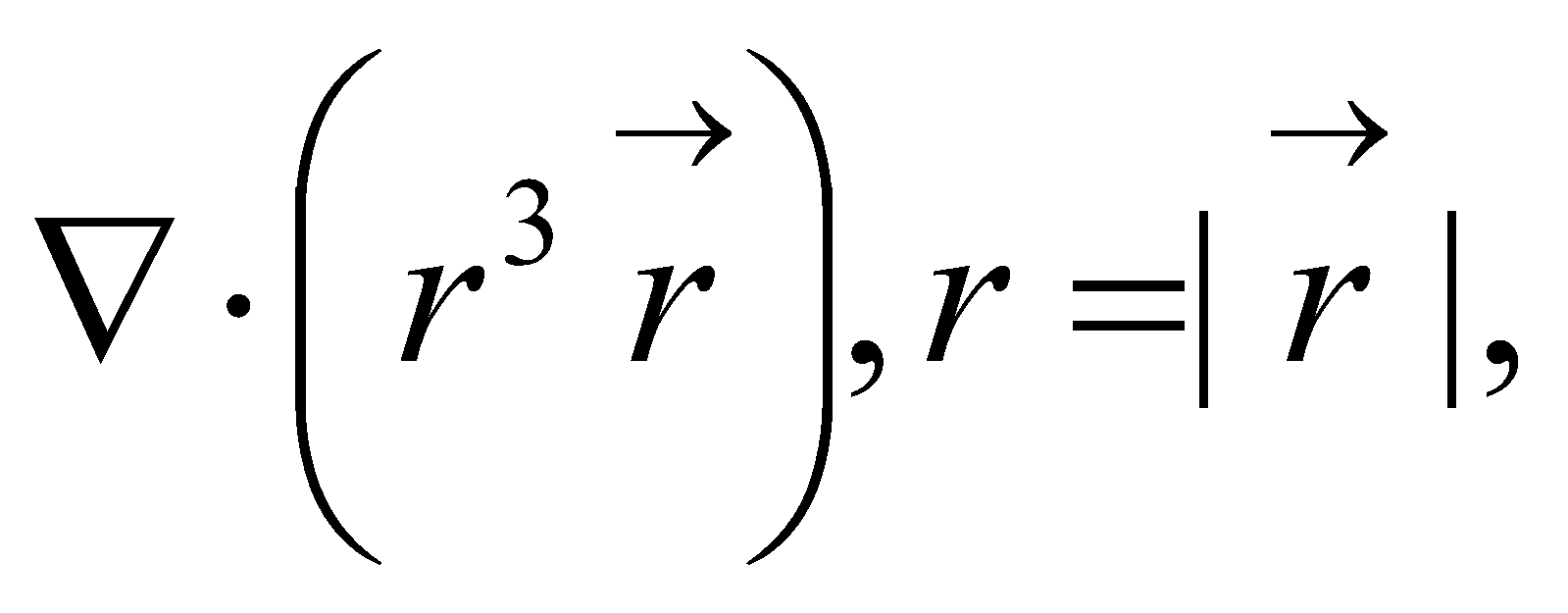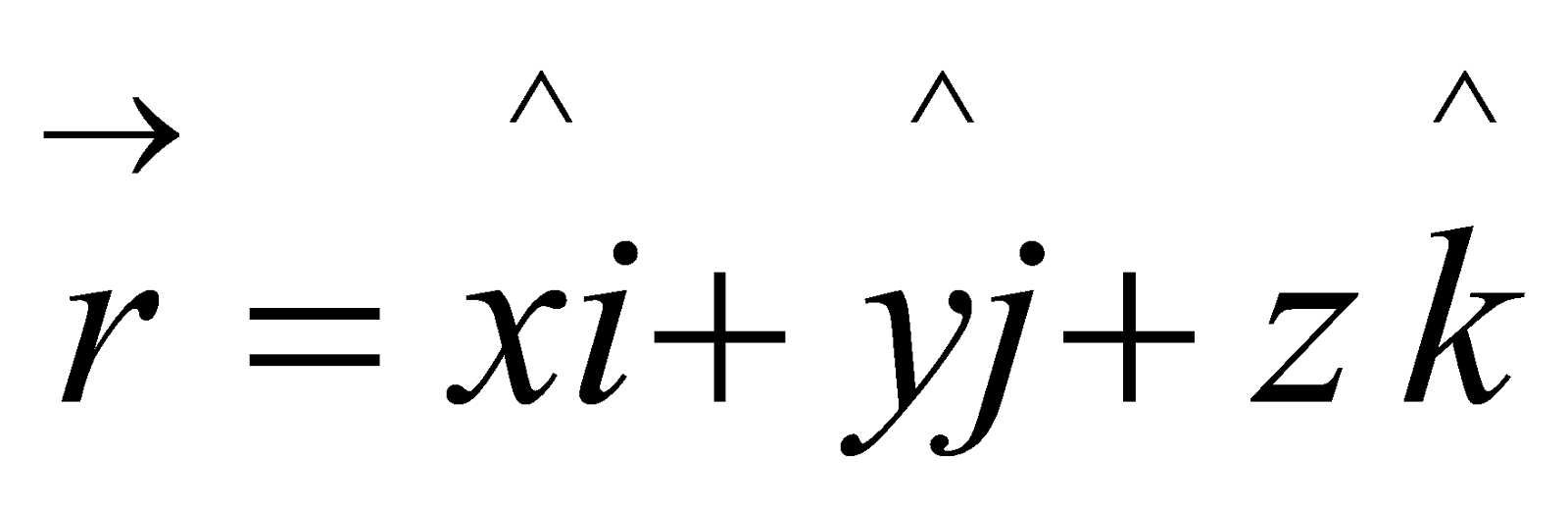3. Show that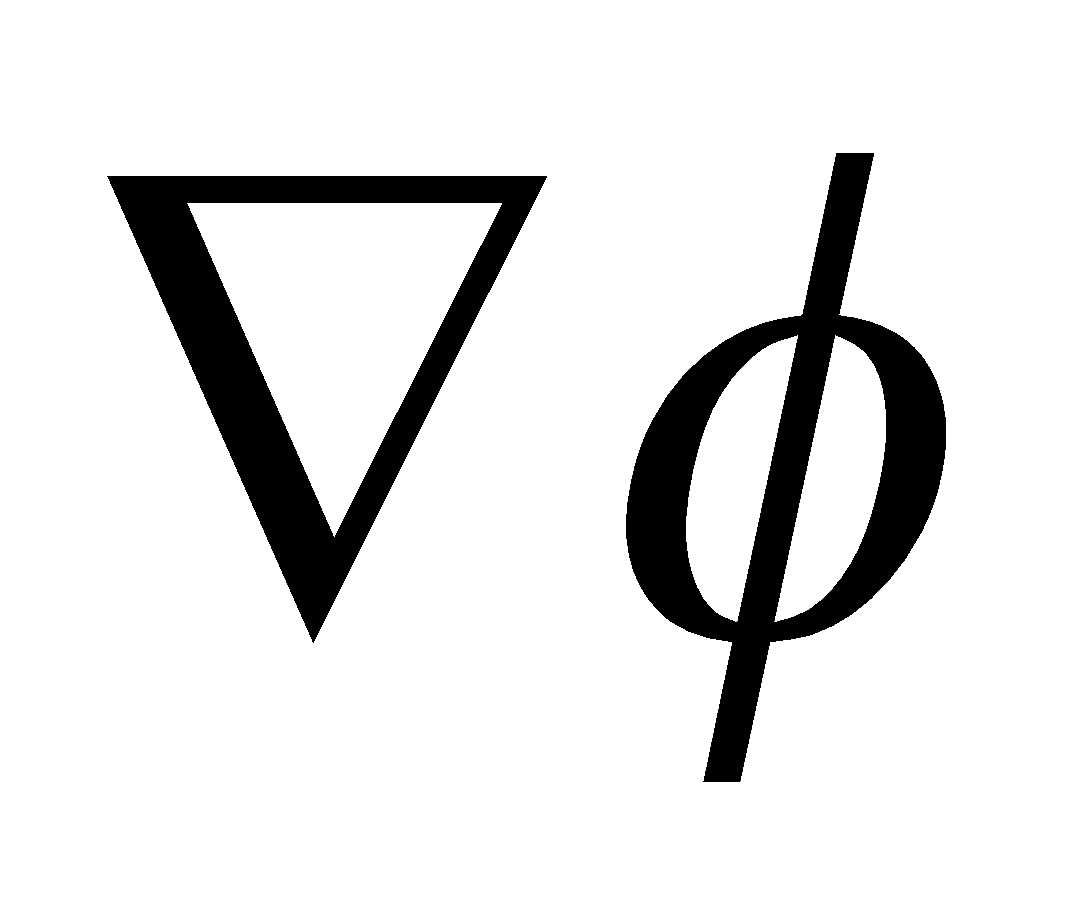is a vector perpendicular to the surface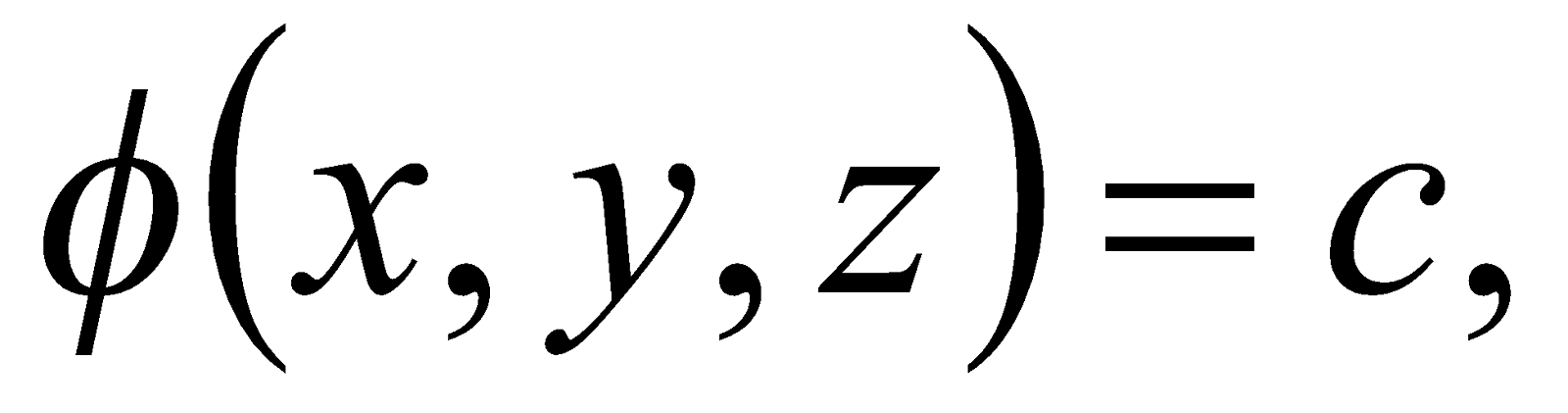where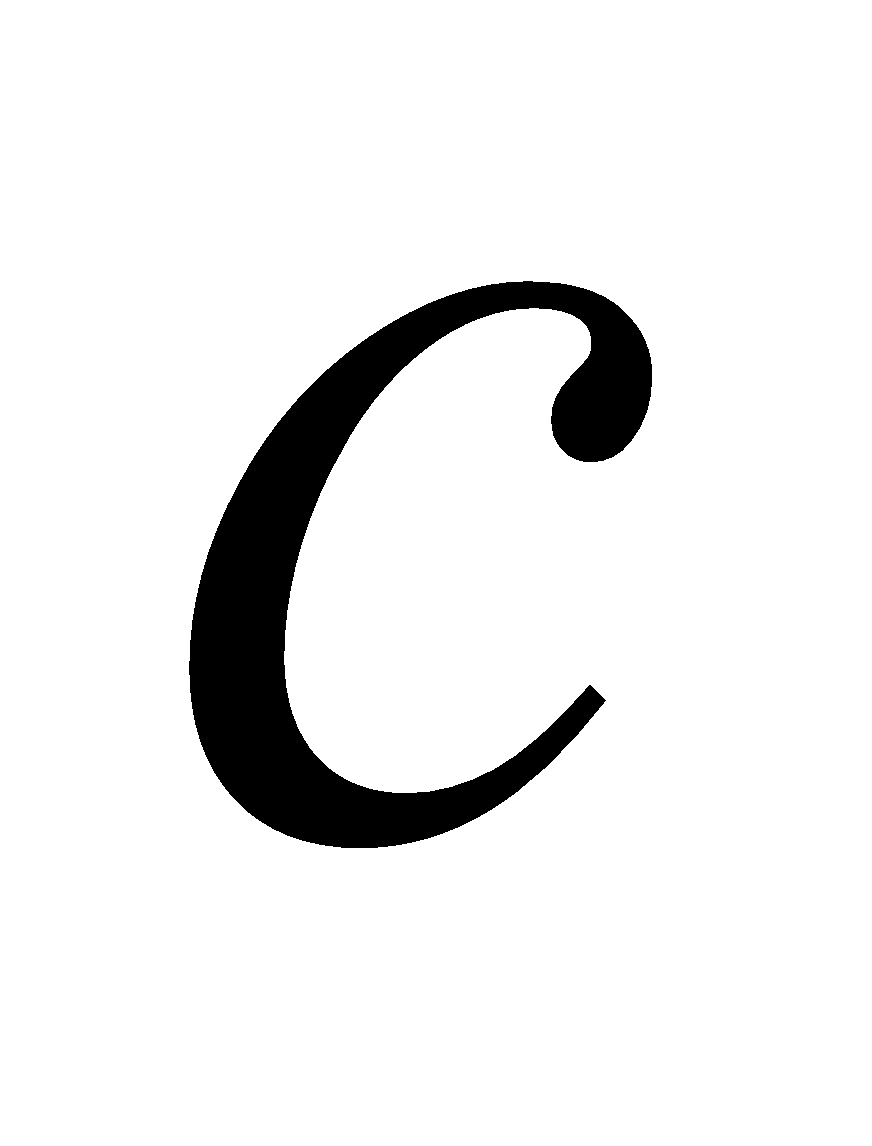is a constant.

***